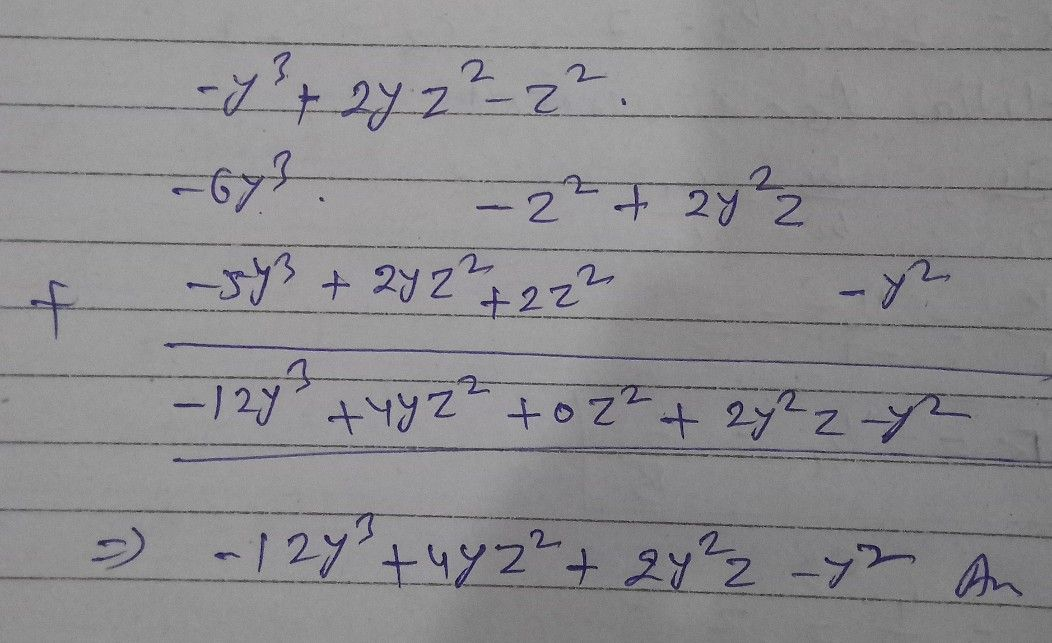Symbol
Problem$-5x^{3}y^{4}+xy,2x^{2}y^{4}+2$ and $7xy-3x^{2}y^{4}$ Cy $-y^{2}+2yz^{2}+2z^{2}-5y^{3}$ $y^{3}+2yz^{2}-2^{2}$ $,-6y^{3}+2\right)^{2}z-z^{2}a$ $and-$ $-c1$
7th-9th grade
Algebra
Question content
Add in algebric expressions
SolutionQanda teacher - PREMLATAStudent
Thank you so much mam